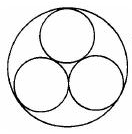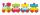# Translate 2

Translate the given phrases to mathematical phrases. Thrice the sum of three fifths and two thirds less one half is what number?

x =  0.3

### Step-by-step explanation:Did you find an error or inaccuracy? Feel free to write us. Thank you!Tips to related online calculators
Need help to calculate sum, simplify or multiply fractions? Try our fraction calculator.

## Related math problems and questions:

• Product and sumWhat is the product of two fourths  and the sum of three halves and four?
• Two thirdsFind two-thirds of the number equal to two-thirds of 99
• Sum of 20Sum of the two numbers is 15 1/6. If one of the numbers is 2 2/3, find the other number.To three-eighths of one third, we add five quarters of one half and multiply the sum by four. How much will we get?
• TranslateTranslate the following mathematical statement into an algebraic expression or equation. 1. Nine less that the quotient of a number and 3 2. The ratio of 3 and the sum of 4 and an unknown number 3. Eighty is the product of 4 and g 4. The sum of x and 18 i
• Number trainThe numbers 1,2,3,4,5,6,7,8 and 9 traveled by train. The train had three cars and each was carrying just three numbers. No. 1 rode in the first carriage, and in the last carriage was all odd numbers. The conductor calculated sum of the numbers in the firs
• Fractions and mixed numerals(a) Convert the following mixed numbers to improper fractions. i. 3 5/8 ii. 7 7/6 (b) Convert the following improper fraction to a mixed number. i. 13/4 ii. 78/5 (c) Simplify these fractions to their lowest terms. i. 36/42 ii. 27/45 2. evaluate the follow
• Sum three fractionsWork out the sum of 1/4, 1/5 and 3/10.
• Math homeworkIt took Jose two-thirds of an hour to complete his math homework on Monday, three-fourths of an hour on Tuesday, any two- fifths of an hour on Wednesday. How many hours did it take Jose to complete his homework altogether?
• Complicated sum minus productWhat must be subtracted from the sum of 3/8 and 5/16 to get difference equal to the product of 5/8 and 3/16?
• ExpressionsLet k represent an unknown number, express the following expressions: 1. The sum of the number n and two 2. The quotient of the numbers n and nine 3. Twice the number n 4. The difference between nine and the number n 5. Nine less than the number n
• Unknown number 16My number's tens is three times more then ones My number's ones is twice the number of thousands and my number's hundreds is half the number of of tens. I have two ones. which number am I?
• Two numbersThe sum of two numbers is 1. Identify this two numbers if you know that the half of first is equal to the third of second number.What is the sum of 2/3 and 3/10?Two-thirds of a number is negative six. Find the number.The sum of 3 mixed numbers is 20 13/15. two of the numbers are 6 1/3 and 7 5/6. what is the third number?What is the value of x in the proportion 2 and one-fourth over x = 1 and one-half over 3 and three-fifths? 2 and two-fifths 5 and two-fifths 8 and 1 over 10 12 and 3 over 20